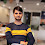Impact-Site-Verification: dbe48ff9-4514-40fe-8cc0-70131430799e

## Search This Blog

MATLAB PROGRAM:

% Romberg integration algorithm
% Find the integral of y=sin(x) from 0 to pi.

f = @(x) sin(x);
a = input('Enter lower limit, a:  ');
b = input('Enter upper limit, b:  ');
n = input('Enter no. of subintervals, n:  ');

h = b-a;
r = zeros(2,n+1);
r(1,1) = (f(a)+f(b))/2*h;
fprintf('\nRomberg integration table:\n');
fprintf('\n %11.8f\n\n', r(1,1));

for i = 2:n
sum = 0;
for k = 1:2^(i-2)
sum = sum+f(a+(k-0.5)*h);
end
r(2,1) = (r(1,1)+h*sum)/2;

for j = 2:i
l = 2^(2*(j-1));
r(2,j) = r(2,j-1)+(r(2,j-1)-r(1,j-1))/(l-1);
end

for k = 1:i
fprintf(' %11.8f',r(2,k));
end

fprintf('\n\n');
h = h/2;
for j = 1:i
r(1,j) = r(2,j);
end
end

OutPut:

Enter lower limit, a:  0

Enter upper limit, b:  pi
Enter no. of subintervals, n:  6

Romberg integration table:

0.00000000

1.57079633  2.09439510

1.89611890  2.00455975  1.99857073

1.97423160  2.00026917  1.99998313  2.00000555

1.99357034  2.00001659  1.99999975  2.00000002  1.99999999

1.99839336  2.00000103  2.00000000  2.00000000  2.00000000  2.00000000

>>

1.Share me the program

1.2.3.